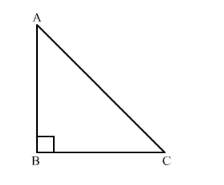# A guy wire attached to a vertical pole of height 18 m is 24 m long has a stake

Question:

A guy wire attached to a vertical pole of height 18 m is 24 m long has a stake attached to the other end. How far from the base of pole should the stake be driven so that the wire will be taut?

Solution:

We will draw the figure from the given information as below,Let AB be the vertical pole of length 18 m and let the stake be at the point C so the wire will be taut.

Therefore, we have $A B=18 \mathrm{~m}, A C=24 \mathrm{~m}$ and we have to find $\mathrm{BC}$.

Now we will use Pythagoras theorem,

$A C^{2}=A B^{2}+B C^{2}$

Let us substitute the values we get,

$24^{2}=18^{2}+B C^{2}$

$576=324+B C^{2}$

Subtracting 324 from both sides of the equation we get,

$B C^{2}=576-324$

$B C^{2}=252$

We can rewrite the 252 as $36 \times 7$, therefore, our equation becomes,

$B C^{2}=36 \times 7$

Now we will take the square root,

$B C=6 \times \sqrt{7}$

Therefore, the stake should be $6 \sqrt{7} \mathrm{~m}$ far from the base of the pole so that the wire will be taut.